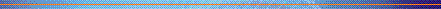Chapter 9

# Models of the System9.1 Using the model-oriented approach with the example graphics program described in Section 9.2.3, specify the move operation as a schema which acts on the currently selected objects. Is the operation you have defined cumulative (two successive moves can be done as one move which is the sum of the other two) or is it 'forgetful?' Discuss the implications of the framing problem in your definition.

###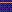answer

Students may not have a great familiarity with the Z notation used in this textbook, especially if they do not have a computer science background which emphasizes formal specification. Nevertheless, we will provide a model answer to this exercise in the Z notation and leave it to the instructor to decide whether an alternative language can be used to express the same ideas.

To accommodate the specification of the move operation, we will first need a function which allows us to add two coordinate points together. We just provide the declaration of this function here.

```
|  _ + _: PointPointPoint
```

The motivation for this function is so that we can define a move operation with a single argument, called delta? below, which will specify how far to move the centres of every selected shape. This approach, using relative movements, will allow for two consecutive moves to be defined with the overall movement being the sum of the individual moves. Another approach (which would be quite cumbersome to define for a set of selected objects) would be to specify the move operation as an absolute operation, the argument(s) to the move explicitly indicating the new value for the centre(s) of each selected shape. This operation definition would not be cumulative.

Initially, we might want to define the move operation simply to update the centre position of all selected shapes.

```
___Move___________________________________________________________
|  State
|  State'
|  delta? : Point
|____________________
|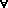id : selectedshapes'(id).centre = shapes(id).centre + delta?
|________________________________________________________________________
```

There are a number of details in this defined operation which are left (dangerously) open to interpretation. Many of these are framing conditions on the Move operation. For example, what happens to shapes which are not selected? The normal intuition on this operation would be that they stay put, but the above definition actually allows them to change in any way -- including the possibility of disappearing altogether. To ensure that unselected objects stay the same as they were before the operation, we would have to add a predicate to the definition.

```
___Move___________________________________________________________
|  State
|  State'
|  delta? : Point
|____________________
|id : selectedshapes'(id).centre = shapes(id).centre + delta?
|id : (dom shapes - selected)shapes'(id) = shapes(id)
|________________________________________________________________________
```

We also need to explicitly state that no new shapes are introduced into the system, i.e., that the domain of shapes remains the same.

```
___Move___________________________________________________________
|  State
|  State'
|  delta? : Point
|____________________
|id : selectedshapes'(id).centre = shapes(id).centre + delta?
|id : (dom shapes - selected)shapes'(id) = shapes(id).centre
|  dom shapes' = dom shapes
|________________________________________________________________________
```

For those familiar with Z, it is possible to collapse the last three predicates in the above schema to one predicate using functional overriding from the basic Z mathematical toolkit (see Spivey ).

Another framing issue which has impact on the interactive style of this graphics system is specifying what happens to the selected set of shapes after the move operation. Our current definition says nothing about this. Two clear options are whether the set of selected shapes remains the same or becomes the empty set (no selected objects). For reasons of compositionality (for defining consecutive move operations or consecutive operations targeted at the same set of shapes) we choose the former option, and specify this below.

```
___Move___________________________________________________________
|  State
|  State'
|  delta? : Point
|____________________
|id : selectedshapes'(id).centre = shapes(id).centre + delta?
|id : (dom shapes - selected)shapes'(id) = shapes(id)
|  dom shapes' = dom shapes
|  selected' = selected
|________________________________________________________________________
```

[Note: Experienced Z users would probably choose the functional overriding operator (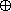) to more concisely express the first three predicates above.]9.2 Write a similar schema for RESIZE. It should have the following informal semantics. The width and height attributes are of the shapes bounding box. The resize operation should make one corner of the bounding box be at the current mouse position (supplied as the argument current_pos?).

Hint: the width of a box is twice the difference between the x coordinate of the centre and the x coordinate of any corner:

wid = 2| centre.x - corner.x |

###answer

Strictly speaking, there are some errors in the Z usage for the hint in this exercise. The type Point is not a schema type, and so it is not meaningful to use schema selection, as in expressions like centre.x and corner.x. The alert Z student should pick up on this error. However, the spirit of the hint is still valid. To make things legal in Z, we can change the declaration of Point as follows:

```
___Point____________________________________________________________
|  x,y :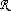|__________________________________________________________________________
```

To best specify this resizing operation, we will require to explicitly model the bounding box of a shape. We introduce a type, called BoundingBox which will represent the corners of the surrounding box which defines the extent of a shape. In fact, in Figure 9.1, we have drawn the bounding box for the circle but we have not made that information explicit in the specification in the text of this chapter. We omit details in this sample answer on 'rectangular' constraints for the corners of the bounding box (e.g., that upper left and right corners have the same y-coordinate values but the x-coordinate of the upper left corner is strictly less than the x-coordinate of the upper right corner, etc.)

```
___BoundingBox______________________________________________________
|  upperleft, upperright,
|  lowerleft, lowerright : Point
|___________________________________________
|  upperleft.xupperright.x
|  upperleft.y = upperright.y
|
|  ...
|__________________________________________________________________________
```

We can add the bounding box as information known about shapes, by extending the definition of Shape. A constraint on the bounding box, motivated by the hint to this exercise, is that its width and height are the same as the width and height of the shape. In addition, the centre coordinates are related to the width and height. Note below that we use the names height and width in the definition of Shape to increase its readability.

```
___Shape______________________________________________________
|  type : Shape_type
|  width :|  height :|  centre : Point
|  bb : BoundingBox
|___________________________________________
|  width = 2(centre.x - bb.upperleft.x)
|  height = 2(centre.y - bb.upperleft.y)
|  width = (bb.upperleft.x - bb.upperright.x)
|  height = (bb.upperleft.y - bb.lowerleft.y)
|__________________________________________________________________________
```

Resizing can be viewed as changing the size of the bounding box. At a relatively abstract level, we can simply specify the resizing operation with a single argument indicating the new bounding box for the selected shape. A precondition for the operation is that there is only one selected shape.

```
___Resize______________________________________________________
|  State
|  State'
|  newbb? : BoundingBox
|___________________________________________
|  #selected = 1
|id : selectedshapes'(id).bb = newbb?
|  forallid : (dom shapes - selected)shapes'(id) = shapes(id)
|  dom shapes' = dom shapes
|  selected' = selected
|__________________________________________________________________________
```9.3 In Section 9.2.5, we said that the specification Algebraic-draw could be extended to say that a move, resize or unselect after a delete has no effect. The axiom for unselect looks like this:

(9) unselect(delete(g)) = delete(g)

Write two more axioms (10) and (11) which say the same about move and resize. Now use axioms (4) and (5) to show that (9) implies both your new axioms.

###answer

(9) unselect(delete(st)) = delete(st)

(10) move(p,delete(st)) = delete(st)

(11) resize(p,delete(st)) = delete(st)

Axioms 4 and 5 are:

(4) move(p,unselect(st)) = unselect(g)

(5) resize(p,unselect(st)) = unselect(g)

To show that Axiom 10 is derivable from Axioms 4 and 9 we can argue as follows:

move(p,delete(st))
 = move(p,unselect(delete(st)) by Axiom 9 = unselect(delete(st)) by Axiom 4 = delete(st) by Axiom 9

A similar argument shows that Axiom 11 is derivable from Axioms 5 and 9.9.4 Imagine a calculator, normal except it displays A for 0, B for 1, up to J for 9. So the number 372 would appear as DHC. Does this affect the formal transparency of the calculator? Should it?

###answer

Formally, this would not affect the transparency property of a calculator. Any function from display to effect that held for the numbers would also hold for their alphabetic counterparts by just composing it with the code A for 0, B for 1, up to J for 9. Of course, most people would probably find the encoded calculator quite a bit more difficult to use than one that used the normal ten digits. The important lesson here is that the formal expression of the transparency property does not indicate how difficult it is for a human to determine the function between display and effect. The formal expression just requires that there be some function. In practice, human users would probably judge the encoded calculator as unpredictable (that is, not transparent). The moral is that even though we can capture a usability property formally this does not mean that we have captured the entire intent of the property. The transparency property would have to be augmented in order to measure the complexity, from the user's perspective, of the function between display and effect.9.5 Can you suggest any improvements to the screen button feedback problem discussed in Section 9.4.5 that would distinguish at the interface between the two cases of hitting or missing the button? Is there any guarantee with your solution that the user will notice the distinction?

###answer

One fix for the button feedback problem would be to have the function attached to the button be invoked when the mousekey is pressed down, rather than up. But this solution would not work for functions invoked on pop-up menus. Another possibility is to have some visible or audible feedback from the button associated with the invocation of the function on the mousekey release. This solution does not guarantee that the user notices the added effect unless their attention is focused on the added visual or aural effect. Another possibility, subtly different from the last suggestion, would be to associate the addition verbal or aural cue to the error case, when the mouse accidentally slips off the button between the press and release of the mousekey.## Worked exercises

The following appears as a worked exercise in Chapter 9:

• A two-function calculator has the following buttons 0-9, +, - and =. These would comprise the command set (C) of a PIE model of the calculator. The state (E) would consist of three components: the two current number being entered, the last number (to be operated on) and the pending operation (+ or -). The display (D) is simply a signed number. For this example, ignore the result.

Write down (semi-formally) the doit function updating the state for each user command and the display function relating the state (E) to the current display (D). To check your definitions: what does the display have on it after the user has entered '2 + 3 +'? Most calculators would show 5, does yours?

Consider the displays after the sequences '2 + 2' '2 +' and the effect on each of the additional user input of 3. Does the calculator satisfy the transparency property? [page 360]

 HCI 2e home page || changes and additions || resources || search || contents || authors || ordering feedback to feedback@hcibook.com http://www.hcibook.com/hcibook/ designed and hosted by hiraeth mixed media webmaster@hiraeth.com